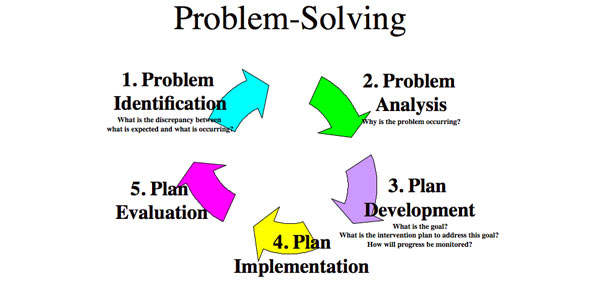5 Questions | Total Attempts: 48SettingsComplete the following questions

Related Topics
• 1.
Use factoring to solve 3x2 – 13x – 10 = 0
• A.

X = 3/2 and x = 5

• B.

X = 2/3 and x = –5

• C.

X = –2/3 and x = –5

• D.

X = –2/3 and x = 5

• 2.
A skateboard company models its profit with the function P(x) = –2x2 + 13x – 15, where x of the number, in thousands that the company sells, and P(x) is the profit in tens of thousands of dollars.  How many skateboards must the company sell to break even? Use factoring to solve
• A.

At 1500 and 5000 skateboards

• B.

At 150 and 500 skateboards

• C.

At 1.5 and 5 skateboards

• D.

At 1500 and 50 000 skateboards

• 3.
Which table of values could be used to solve –2x2 – 5x + 3 = 0?
• A.

Table A

• B.

Table B

• C.

Table C

• D.

Table D

• 4.
Kevin threw a ball into the air.  The height of the ball is modelled by the function h(t) = –5t2+35t, where h(t) is the height of the ball in metres and t is the time in seconds.  When does the ball hit the ground?
• A.

1s

• B.

3.5s

• C.

7s

• D.

61.25s

• 5.
Use the graph of the function below to solve the corresponding quadratic equation.
• A.

X = –16 and x = 0

• B.

X = 0.8 and x = –4

• C.

X = 0.8 and x = 0

• D.

X = 4 and x = 1.25